Saturday, October 6, 2018

Flare-On 5 CTF - Challenge 12 Writeup

Flare-on was a blast this year ! All challenges were great but I enjoyed solving the last one the most, although it was somewhat frustrating.

Due to my tight schedule, I won't go over all the details involved in solving the challenge. But I'll do my best to paint a complete picture of what's going on and how I approached the problem.

We start we a floppy disk image that you can download from here (PW : infected) :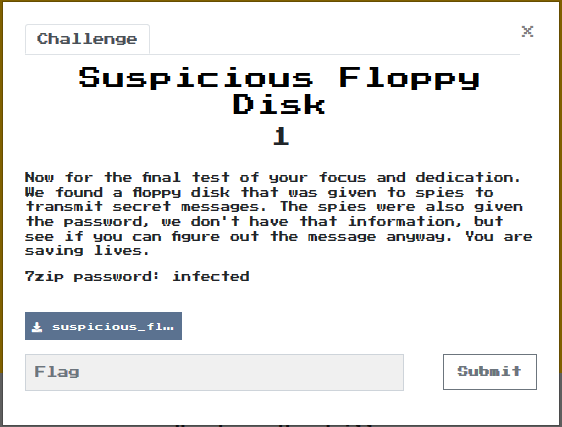When we boot the floppy using bochs, we see that it automatically executes infohelp.exe that asks us for a password.
Entering an arbitrary password, the message "Welcome to FLARE..." prints slowly on the screen and the password checks are performed.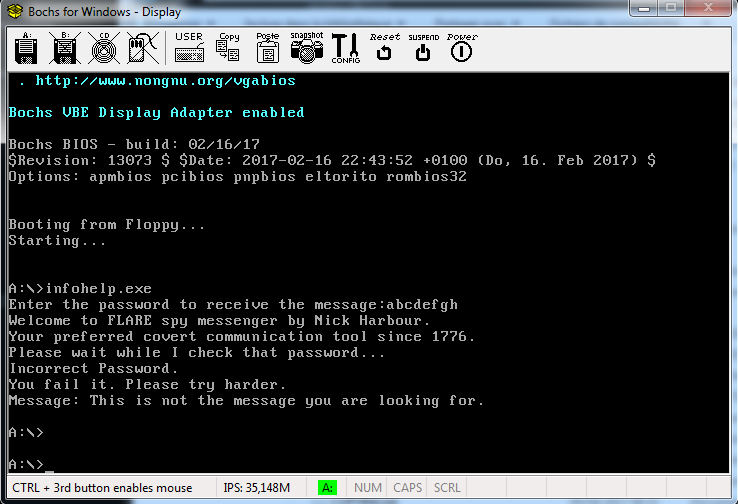What I did after this, is I mounted the floppy on my Ubuntu virtual machine and extracted the files in bold.

souhail@ubuntu:/mnt/floppy\$ ls
AUTOEXEC.BAT  EGA2.CPI      IO.SYS        KEYBRD3.SYS  MODE.COM
COMMAND.COM   EGA3.CPI      KEYB.COM      KEYBRD4.SYS  MSDOS.SYS
CONFIG.SYS    EGA.CPI       KEYBOARD.SYS  key.dat      TMP.DAT
DISPLAY.SYS   infohelp.exe  KEYBRD2.SYS   message.dat
souhail@ubuntu:/mnt/floppy\$

Both key.dat and message.dat contain nothing interesting. However, TMP.DAT appeared to contain the welcome message we see after typing the password and some funny strings like : "NICK RULES" and "BE SURE TO DRINK YOUR OVALTINE".

What I did next is I threw infohelp.exe into IDA to examine its behavior. To my surprise, I found that it does nothing but writes the supplied password to key.dat and then reads the contents of message.dat and prints them to the screen.

Here I thought that there should be some hooks involved that redirect execution to somewhere else when message.dat is opened or read. To confirm my suspicions, I executed the "type" command on message.dat; Lo and behold, the password check is performed.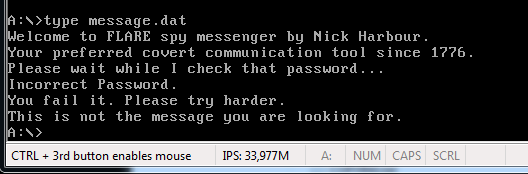Next, I opened TMP.DAT in IDA and found that it contains some code that seems to be our hook. So I attached IDA to bochs and started debugging.

To locate the hook within memory, I took advantage of the fact that the message is printed in a slow fashion so what I did is pause execution while stuff was still printing. I found myself in a loop implementing the subleq VM.

The caller supplies a pointer to the bytecode, its size, and the offset to the instruction where execution should start.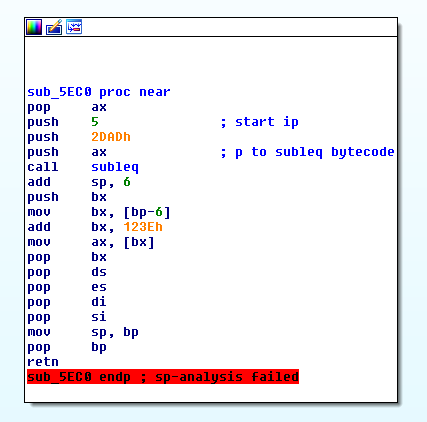Each instruction is 6 bytes and has the following format :

struct inst {
WORD src_index;
WORD dest_index;
WORD branch_index;
};

The type of the subleq bytecode array is WORD*, so the VM views that its instruction size is 3 while it is 6 actually. This realization comes in handy when building an IDA processor module for the subleq.

As I did with last year's binary, I re-implemented the subleq VM with C to output each executed instruction to a file. However, I had an impossible-to-analyze file with over 1 GB. So what I did, is only print the subleq for the instructions that perform the password checks; That way I had a 30 MB-ish file that I could examine looking for patterns.

The way I had the emulated instructions printed was the following :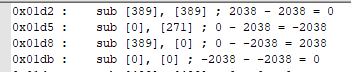IP : sub [dest_index], [src_index] ; subtraction = result

The only thing that was visible on the fly is that the subleq starts by doing some initialization in the beginning and then enters a loop that keeps executing until the end. Here where suspicions of a second VM started to arise in my mind (OMG !).

I tried to locate the password characters inside the subleq and tried to figure out what operations were done on them but it was not clear at all.

I also did some text diffing between iterations and saw that the code was modifying itself. In these instances, the self-modification was done in order to dereference VM pointers and use their values as indexes in subsequent operations.

So, what I decided to do here is write a very minimal processor module that would allow me to view the subleq in a neat graph.

The module's source code is available here.

The file I extracted contains bytecode starting from IP : 0x5. So here's how you load it into IDA :

- Choose the subleq bytecode processor module and make sure to disable auto-analysis. It ruins everything when enabled.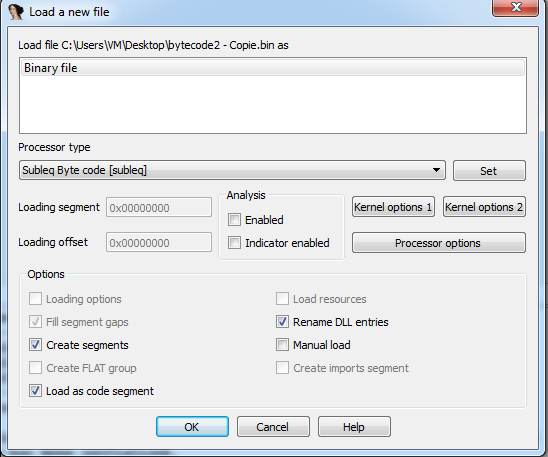-  Change the ROM start address to 0xA and the input file loading address to the same : 0x5 * sizeof(WORD) == 0xA.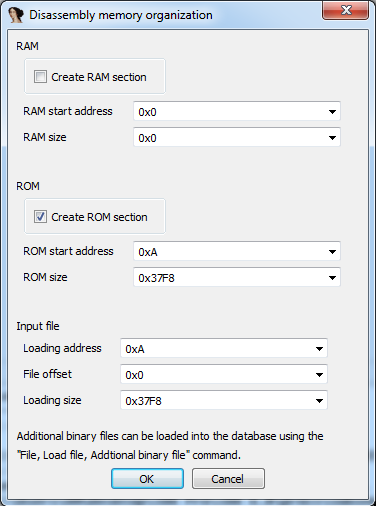The bytecode will be loaded without being analyzed.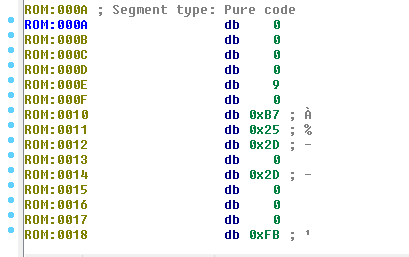- Press 'P' at 0xA to convert into code and automatically into a function. You should have a beautiful graph as a result.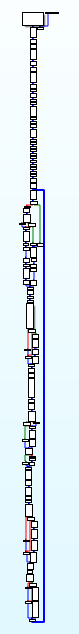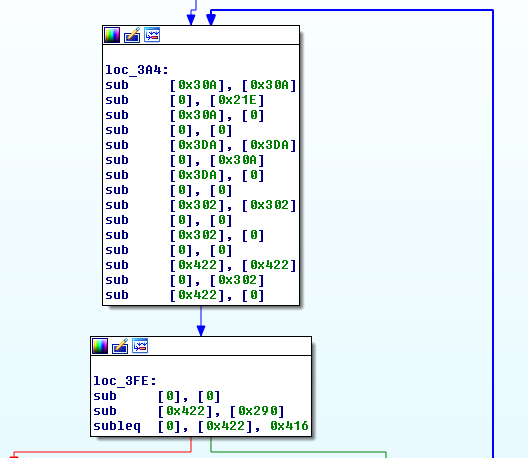Well, it is not quite as beautiful as you might think, since we still have to deal with self-modifying code (knowing what exactly is modified) and also understanding the code as a whole.

It is quite hard to understand what subleq does by only reading "subleq" instructions, so the next thing that came to mind is to convert the subleq to MOV and ADD instructions without wasting too much time.

IDAPYTHON TO THE RESCUE !

I wrote a minimal script that looks for ADD and MOV patterns in the subleq and comments these instructions. First of all, the script intentionally skips the instructions that will be self-modified and doesn't comment the SUB since it's already there.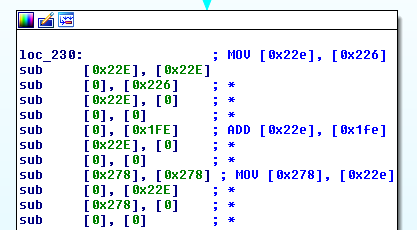More understandable ... still, not so much.

So what I did next is decompile this manually into C and then simplify the code.

______________________________________________________________
WORD* ptr = vm_code;

int i = 0;

while ( ptr < 0x4B6E )
{
WORD op_addr = ptr[ ptr ];
WORD res;

if ( op_addr == -2 )
{
break; //Exit
}

if ( op_addr == -1 )
{
ptr++;
}
else
{
res = ptr[op_addr] - ptr; //SUB op, ACCUM

ptr = res; //ACCUM

if ( op_addr != 2 )
{
}

if ( res < 0 )
{
ptr++;
}

ptr++;
}

if ( ptr == 1 )
{
printf("%c", ptr);
ptr = ptr = 0;
}
if ( ptr == 1 )
{
ptr = 0;
scanf("%c", &ptr);
}
}
______________________________________________________________

So it is indeed another VM interpreted by the subleq. The nature of the VM was unknown to me until later when someone told me that it was RSSB. But I was able, however, to solve the challenge without needing that information.

Now, this RSSB VM executes bytecode that starts at offset 0xFEC from the start of the subleq or at offset 0x1250 of the TMP.DAT file.

If you dumped the bytecode from memory as I did, you'd find that the password you typed was written inside the RSSB VM at offset 0x21C (circled in red).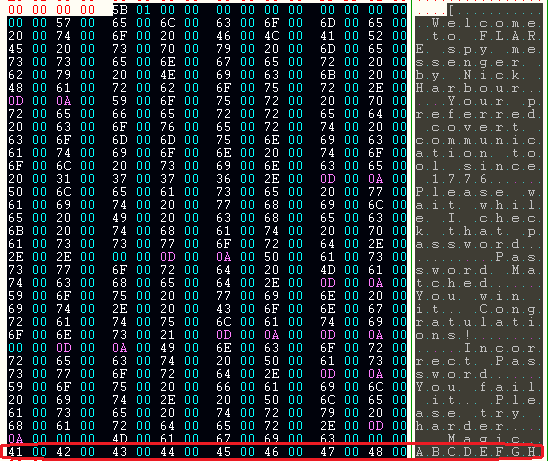So far so good. I copied the whole RSSB bytecode and added it as an array and modified the C emulator code to print the "sub" instructions while executing the VM; the same way I did with the subleq.

The result looked like this :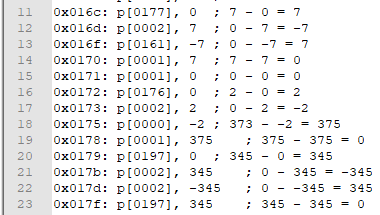IP : p[dest_index], ACCUM ; operation

Reading the code, I found out that a sum is calculated for the characters in the password. In addition to that, the number of characters in the password must be 64. I knew that by examining a variable that gets decremented from 64 at each iteration of the sum calculation.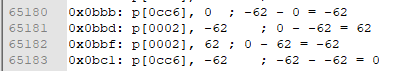For information, the sum is stored at : p[0b47].

So I patched the memory to store a 64 byte string and then I looked up where the first character of the input was read apart from where the sum was calculated. I performed a search for [010e] ( 0x21C / 2 == 0x010E).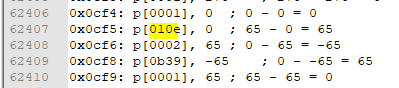65 in dec == 0x41 in hex

Long story short, the algorithm works in a loop, in each iteration two characters of the password are used to calculate a value. The sum of all characters is then added to that value as shown in the figure below (sum : in red, value : in green).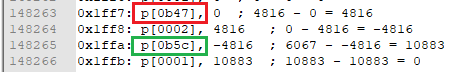A few instructions later, a hardcoded value at [0a79] is subtracted from the resulting value of the previous operation.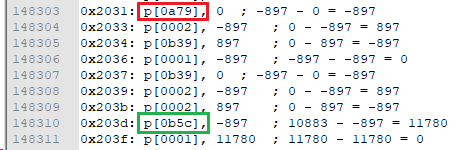We can see that the resulting value of the next two characters for example is compared against the next hardcoded value at [0a7a] and so on until the 30th character.

So, we have a password of 64 bytes but from which only the first 30 bytes are checked !
Let's leave that aside for now and ask : what makes a check correct ? Maybe the result of the subtraction must be zero ?

I quickly added a check onto my C emulator that did the following :

if ( ptr == 0x203d ) //IP of the check, see figure above
{
res = 0;
}

This will simply patch the result to zero in the check at 0x203d. I ran the program and it showed that the password was correct, so I knew I was on the right path.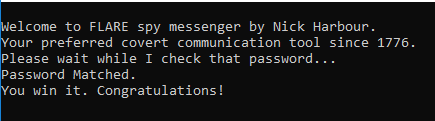I also observed (based on a single test case) that in each iteration the calculated value depends on the position of the two characters. So even if we have the same two characters at different places the calculated value will be different.

Solution : Here I am going to describe how I solved the challenge during the CTF. Surely this is not the best solution out there, but I'd like to show my line of thought and how I came to solve this level.

We know that the same sum is used in all the operations, and that there can be only one sum (or a handful as we'll get to see later) that will satisfy all the checks.

We can run a bruteforce attack where we let the VM calculate the value for two given characters (by patching the characters at run-time) then run the check on all possible sums (by patching the sum at run-time too). The first check will give us a lot of sums that we'll use to bruteforce the second check. In its turn, this check will filter out invalid sums that we'll eliminate from the third check and so on until we do all 15 of them. (30 characters / 2 characters per check == 15 checks).

At the end, we'll get the valid sums from which we can easily deduce the characters that generated them in each check.

The problem I had with this approach was performance. For each combination of two characters, and for each sum, I was running the VM all over again which, if I left like that, would take a huge amount of time : printing the welcome message, calculating the sum for junk characters ... over and over again each time !

What I ended up doing is "snapshotting" the VM in multiple states.

Snapshot 1 : Where the first character of the two is read (depending on the iteration we're in).
Snapshot 2 : For each two characters, take a snapshot after the value that depends on both of them is calculated and right at the address where the sum is read and added to the calculated value (IP == 0x1ff7).

The optimization here is that we execute what we only need to execute and nothing more. We patch the two characters by the ones we're currently bruteforcing at Snapshot 1 and then after the value is calculated for those two we take Snapshot 2 and we only get to patch the sum. When each iteration is over, we re-initialize the snapshots to their original states.

Here's the overall algorithm involved in pseudo-code (this is not C nor anything close) :

sums [xxx] = {...};
new_sums [yyy] = {0};

for ( i = 0; i < 15; i++)
{
memcpy(initial_state_tmp, initial_state);
snapshot_1 = take_snapshot_1(initial_state_tmp, i); //i is passed to go to the corresponding check

for ( c1 = 0x20; c1 <= 0x7F; c1++ )
{
for ( c2 = 0x20; c2 <= 0x7F; c2++)
{
memcpy(snapshot_1_tmp, snapshot_1);
write_characters_to_mem_at_offset(snapshot_1_tmp ,c1 , c2 , i);

snapshot_2 = take_snapshot_2(snapshot_1_tmp);
for ( sum in sums )
{
memcpy(snapshot_2_tmp, snapshot_2);
write_sum_to_mem(snapshot_2_tmp ,sum);
//Execute the subtraction check and return a boolean
if ( execute_check(snapshot_2_tmp) )
{
append(new_sums, sum); //valid sum, append it
}
}
}
}
sums = new_sums;
new_sums = ;
}

print sums;

At the end we'll get the valid sums that resulted in the check being equal to 0.

Here's the full C script implementing this (a bit messy) :

After the 15 checks are done, the script gives us files containing the valid sums that passed each of the checks. We're only interested in the last file (4KB in size) highlighted below :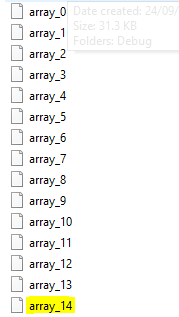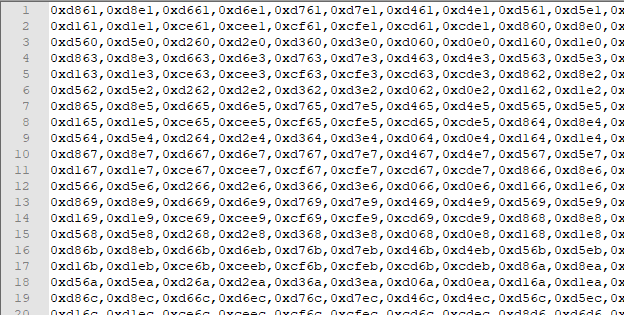Contents of array_14

I actually forgot to include the characters that generated the sum for each check. And I had to do it separately.

This requires some modifications of the code above : we initialize the sums array with the contents of array_14 and for each sum bruteforce the two characters that pass each check. To optimize, I listed the first four characters (two first checks) for each one of these sums.

And one of them was particularly interesting. The sum 0xd15e resulted in these four characters "Av0c".

Running the same script for this single sum while bruteforcing all of the characters gives us the flag :